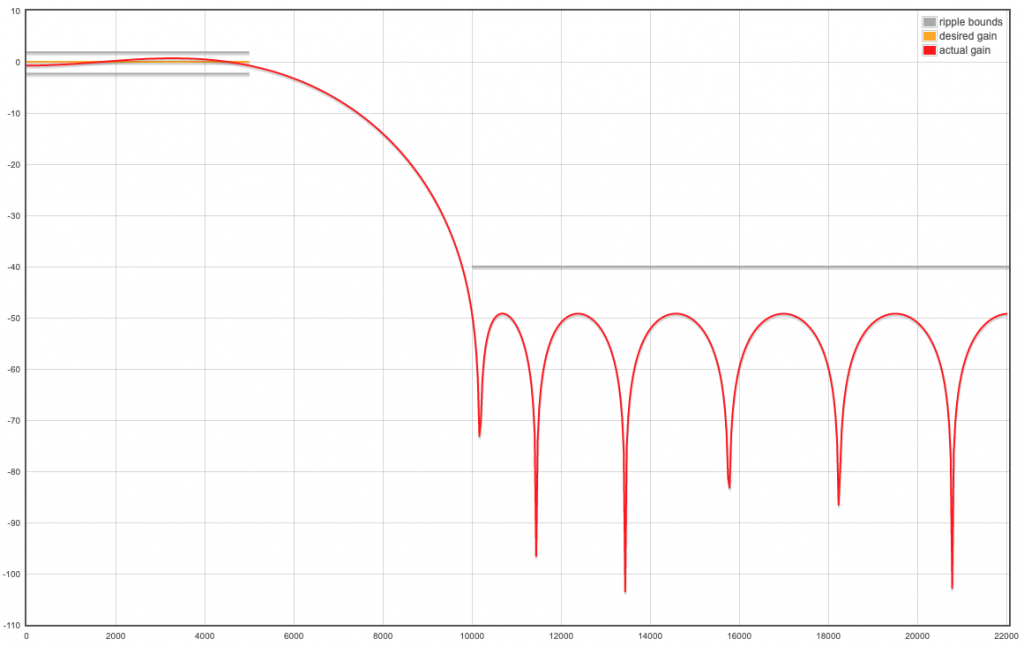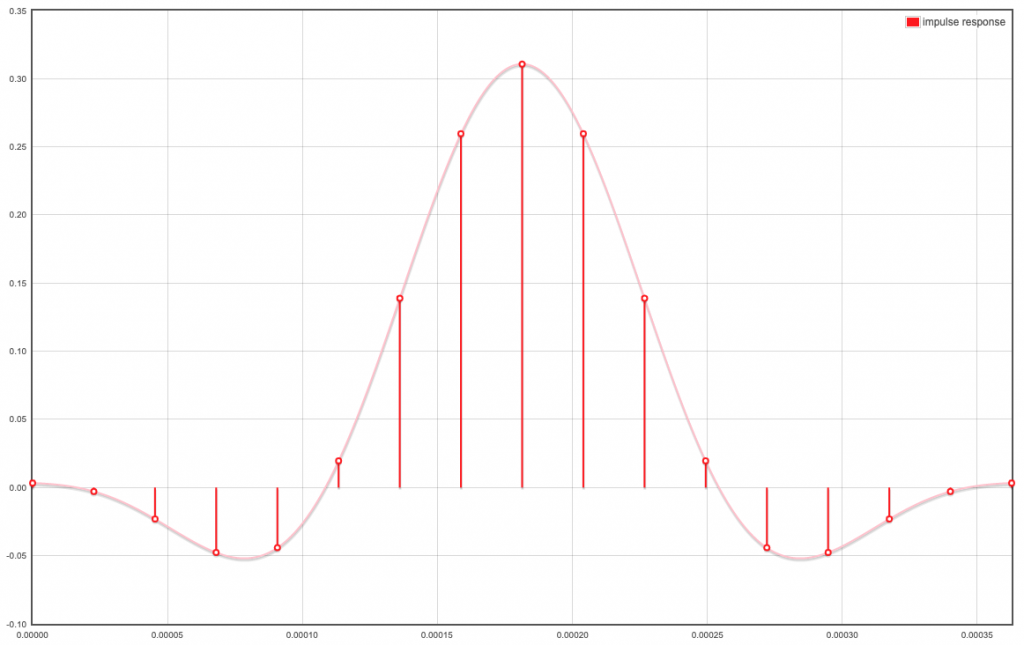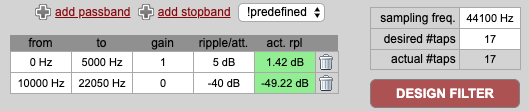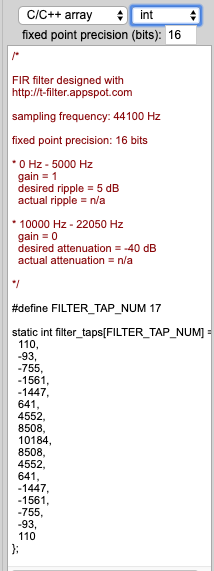# APU Configuration

• @MyAmigo Great! That is one more step to deal with the apu.
For all those without a copy of matlab. At http://t-filter.engineerjs.com/ very similar filter coefficients can be created:TFilter uses int16_t numbers, sdk example apu/init.c uses uint16_t.

• @manageryzy thank you. I thought the common coefficients were there to apply gain. I can confirm whether that is actually used by changing the values and seeing if it affects the performance of the hardware. Speaking of gain... there is a hardware function called audio_bf_set_audio_gain which I assume is adjusted to correct overall gain for the system so it is applied to all frequencies. Does that sound right?

I figured out how to generate a filter in Matlab using just the coefficients so I loaded the 17 coefficients from the BF demo into Matlab (after converting to fixed-point real numbers) and obtained the following impulse reponse (to confirm import)...and the resulting magnitude response...... assuming FS=48kHz. This is helpful as it suggests a Fpass of about 5kHz and an Fstop of about 10kHz if we use MatLab's filterDesigner tool. So I did that. The impulse and magnitude response for an equiripple, low pass, FIR filter with order N=16, Fpass=5kHz and Fstop=10kHz is as follows...This is very close to the filter that was generated using just the demo coefficients so I'm definitely on the right track. I note that the coefficients are about 2x those of the demo and there is an additional ripple "hump" in there but this doesn't look too bad.

I feel like I'm close to a solution but I should be able to reproduce the coefficients from the demo exactly so I would like some feedback on this.

Knowing the specific parameters that were used to determine the demo FIR coefficients would solve my problem.

Thanks... Scott

• @MyAmigo 0x03c3 is just a test value , i don't know why whese code got into drivers ... use your own coef

just generate a fir by matlab filter design tool, use fiter design tool quantilize these coeffs and copy them here

• I figured out what the fixed precision values (from -1 .. +1) are as follows for both the PREV & POST FIR vectors...I might be able to find a Matlab filter to match these coefficients but I'll be guessing so if you can provide a little more info I'll be able to move this further along.

What about the 3rd FIR filter vector?

``````uint16_t fir_common[] = {
0x03c3, 0x03c3, 0x03c3, 0x03c3, 0x03c3, 0x03c3,
0x03c3, 0x03c3, 0x03c3, 0x03c3, 0x03c3, 0x03c3,
0x03c3, 0x03c3, 0x03c3, 0x03c3, 0x03c3};
``````

How is this used? You didn't show it in your earlier data flow diagram.

Thank you for your help. I really appreciate it.

Scott

• Great thanks! Can you provide the Matlab code to do this? That way I don't have to guess which filter command to use.

• signed fixed point number between -1 ~ 1

u can use matlab generate these coeffs

• I tried to answer my own questions about the number format for the cofficients. A signed 16-bit representation of a real number is available with the half-precision floating-point format. Converting the coefficients with this format results in the ones starting with 0xF being very large numbers so this can't be right.

Are there other possible formats for floating point numbers that might be used here? It would be nice to hear from a developer of the APU hardware.

• @manageryzy thank you.

This is helpful but I need to know more about the design of the FIR filters. There are 17 coefficients and I recognize that they are symmetric so that should narrow the options.

There are a variety of methods that may have been used to design a FIR filter:
https://en.wikipedia.org/wiki/Finite_impulse_response

• Window design method
• Frequency Sampling method
• Weighted least squares design
• Parks-McClellan method (also known as the Equiripple, Optimal, or Minimax method)
• Equiripple FIR filters designed using FFT algorithms

Someone must know the type of filters that are built into the hardware so please advise. If I have that information then I can use Matlab to calculate the coefficients for the filter used in the demo and then I will be able to create a filter with different properties.

Having an explaination of the values in the fir_prev_t definition ...

``````uint16_t fir_prev_t[] = {
0x020b, 0x0401, 0xff60, 0xfae2, 0xf860, 0x0022,
0x10e6, 0x22f1, 0x2a98, 0x22f1, 0x10e6, 0x0022,
0xf860, 0xfae2, 0xff60, 0x0401, 0x020b,
};
``````

... would be a big help as well. Are these real-number representations?

I can't move forward without assistance.

• This post is deleted!

• This post is deleted!

• This post is deleted!

• although i can't tell you the details of apu , i can tell you some pipeline about it

+----------+ 　+----------+ 　+------------+ 　+-------+ 　+-----+　+--------------------- + 　+-----+ 　+-----+
| 8ch in | - > | buffer | - > | DAS BF | -> | gain | -> | FIR | -> | DownSample | -> | FIR | -> | FFT |
+----------+　 +----------+ 　+------------+ 　+-------+ 　+------+　+---------------------+ 　+------+　+------+